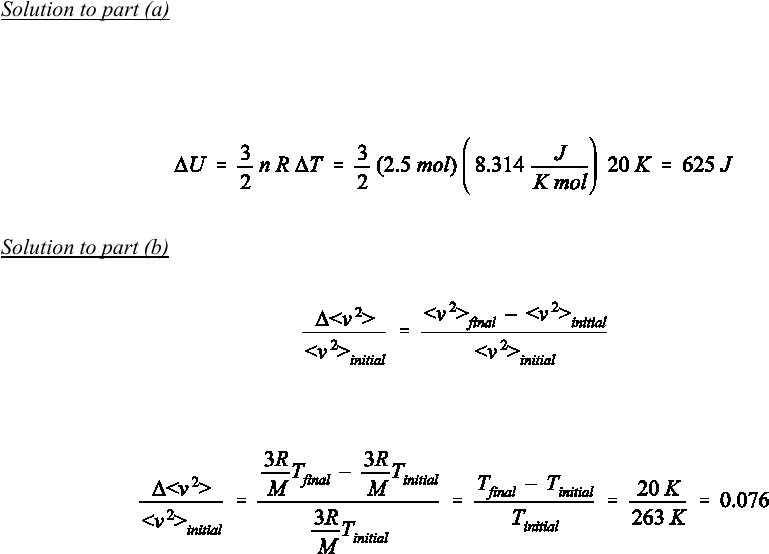# Physics 1028 Lecture 8: Chapter 10

4 views3 pages
School
Western University
Department
Physics
Course
Physics 1028
ProfessorSelection of Even–Numbered Problem Solutions
Chapter 10
P–10.2
Solution to part (a): The argument for the amount of heat is based on the first law of thermodynamics for a
closed system. We know that W = 0 J since the volume in this problem remains constant (no provision is
given to allow for an increase or decrease of the container size). Therefore, U = Q. Finding the increase in
the internal energy allows us to determine the amount of heat required.
For an ideal gas, we use Eq. [8.24] for U with n = (100 g) / (39.95 g/mol) = 2.5 mol. Thus:
(1)
Solution to part (b): Note that the question is not for the root–mean–square speed but for <v²>. The relative
increase of this quantity is defined as:
(2)
i.e., the relative increase is a fraction of the initial value. The result must be multiplied by 100 in order to
express this value in percent. Using Eq. [8.25] we rewrite Eq. :
(3)
This corresponds to a 7.6% increase relative to the initial value.
Unlock document

This preview shows page 1 of the document.
Unlock all 3 pages and 3 million more documents.

## Document Summary

Solution to part (a): the argument for the amount of heat is based on the first law of thermodynamics for a closed system. We know that w = 0 j since the volume in this problem remains constant (no provision is given to allow for an increase or decrease of the container size). Finding the increase in the internal energy allows us to determine the amount of heat required. [8. 24] for u with n = (100 g) / (39. 95 g/mol) = 2. 5 mol. Solution to part (b): note that the question is not for the root mean square speed but for . The relative increase of this quantity is defined as: i. e. , the relative increase is a fraction of the initial value. The result must be multiplied by 100 in order to express this value in percent. This corresponds to a 7. 6% increase relative to the initial value.